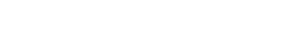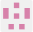BackSolution

by Trapdoor Tech

The air makes no sound!

### Introduction

All information relating to this puzzle, Can you turn up the heat? can be viewed here. The background resources for this puzzle are also linked below.

## See puzzle details on Github

### Solution

This week has the most difficult puzzle that we've ever solved. One must have a clear understanding of the whole STARK proving system in order to work it out. So what is the puzzle? Reading from the code, we know that Alice received an incorrect proof that can still be verified. Our mission is to find out how to generate it. A valid proof should be able to prove a Fibonacci computation trace, on the start and end positions constraints are satisfied.

### The DEEP-FRI Method

We can list the constraints here. Since we are manipulating a set of 2-column state registers, let's denote the 1st column as polynomial $$f_0(x)$$ and the 2nd $$f_1(x)$$. We should have: $$f_0(0)= 0$$ $$f_1(0) = 1$$ $$f_0(15) = 832040$$ $$f_0(x) + f_1(x) = f_0(gx)$$ $$f_1(x) + f_0(gx) = f_1(gx)$$
According to STARK proving system, these constraints should be converted to a algebraic representation. They can be constructed by polynomial division. So we have: $$C_0(x) = \frac{f_0(x) - f_0(0)}{x-g^0} = \frac{f_0(x)}{x-1}$$ $$C_1(x) = \frac{f_1(x) - f_1(0)}{x-g^0} = \frac{f_1(x) -1}{x-1}$$ $$C_2(x) = \frac{f_0(x) - f_0(15)}{x-g^{15}} = \frac{f_0(x)-832040}{x-g^{15}}$$ $$C_3(x) = \frac{f_0(gx) - f_0(x) - f_1(x)}{x^{15}-1 / x-g^{15}}$$ $$C_4(x) = \frac{f_1(gx) - f_0(gx) - f_1(x)}{x^{15}-1 / x-g^{15}}$$
Then we use one composition polynomial to combine all the constraints. We will just paste the formula here: $$\sum_{j=0}^{k-1} C_j(x)(\alpha_jx^{D-D_j}-\beta_j)$$
If the degree of the composition polynomial is less or equal than $$D$$, then we know all the constraints are satisfied. However in STARK proving system, we are not actually checking this composition polynomial. We construct another $$DEEP$$ polynomial to ensure: < br>
* at an random point $$z$$, the composition polynomial and all the trace polynomials $$f_k(x)$$ are evaluated correctly
* the $$DEEP$$ polynomial is a low-degree polynomial, $$D_{deep} < D$$

The $$DEEP$$ polynomial is also a combination of the above polynomials. Here we paste again from the StarkEx article, note that $$H_1(x)$$ , $$H_2(x)$$ are the even/odd parts of the composition polynomial:
$$\sum_{j=0}^{M-1}\alpha_j.\frac{f_k(x)-y_j}{x-zg^a}+\alpha_M.\frac{H_1(x)-H_1(z^2)}{x-z^2}+\alpha_{M+1}.\frac{H_2(x)-H_1(z^2)}{x-z^2}$$
The last question is how to do a low-degree test? Here comes the FRI protocol but we will not explain its details. All you need to know is that we let the prover commit to a polynomial evaluation merkle tree. After commitment, we challenge the prover $$D+1$$ times to get $$D+1$$ polynomial evaluations. Then we interpolate a $$D-1$$ degree polynomial $$f(x)$$ with $$D$$ evaluations $$f(x_d)$$, check if the remaining evaluation is equal to $$f(x_{d+1})$$. If yes then we are almost sure that the original polynomial is a low-degree (less than $$D$$) polynomial. (FRI optimized the challenge process so that we don't actually need to challenge $$D+1$$ times, extremely useful when $$D$$ is huge)

### The Hack

What if we provided an invalid program trace to the verifier? First of all, the constraint polynomial cannot be divided by its divisor, so the quotient polynomial will not be a low-degree polynomial. Therefore, the DEEP polynomial will not be a low-degree polynomial, and it cannot pass the FRI low-degree test, with a high probability, but how high is the high probability?
The number of queries in proof options is set to 1, so we know the verifier only query once for the DEEP polynomial. By once, we mean that verifier only checks at one random point in the LDE domain that the DEEP polynomial is indeed constructed by those trace and constraint polynomials. So if we generate a fake DEEP polynomial $$DEEP_{fake}$$, that at some certain points it's evaluation is equal to the $$DEEP_{real}$$, we have a chance that it can pass the verifier check. Moreover, this $$DEEP_{fake}$$ should have low-degree, otherwise we cannot pass the successive FRI low-degree test.
Now the hack is clear, steps are described as below:

* we generate a fake trace, use the fake trace to compute all the trace polynomials and constraint polynomials. They are of high-degree, but that doesn't matter.
* we generate the composition polynomial, be aware to make sure that the composition polynomial is a combination of those fake polynomials in step 1
* we carefully generate the $$DEEP_{real}$$ polynomial, it can be evaluated by the above polynomials, but is of high degree. As in step 1, it doesn't matter because we are not going to send this polynomial to the verifier.
* we generate a $$DEEP_{fake}$$ polynomial, it evaluate to the same result as $$DEEP_{real}$$ polynomial, but only at some certain points. We can do this by sampling point evaluations from $$DEEP_{real}$$ polynomial, and use Lagrange Interpolation to construct $$DEEP_{fake}$$. The maximum point number sampled is equal to the low-degree that we are aiming for. (If we take more that number of points, we will have a interpolated polynomial whose degree exceeds the low-degree).
* we use $$DEEP_{fake}$$ polynomial to run the FRI protocol. Since the polynomial is of low-degree, the FRI test shall always pass.
* The verifier also use queries to check whether the $$DEEP_{fake}$$ is the $$DEEP$$ polynomial that he expects. Since he only query once, we have a non-negligible succeed chance.

### Puzzle Winners01## wborgeaud

Puzzle completedScore

100002## niooss-ledger

Puzzle completedScore

980.503## Konstantce

Puzzle completedScore

969.3

Lorem ipsum dolor sit amet, consectetur adipiscing elit. Molestie vel vulputate diam condimentum tristique est. Eget gravida sagittis, mi donec est. Amet turpis leo morbi enim nulla lorem sed justo. Tincidunt eu diam pellentesque nibh blandit viverra. Tincidunt pellentesque ultrices ac, sed quis purus ac. Orci adipiscing sapien aenean tincidunt rutrum. Molestie in tortor risus est.Lorem ipsum dolor sit amet, consectetur adipiscing elit. Molestie vel vulputate diam condimentum tristique est. Eget gravida sagittis, mi donec est. Amet turpis leo morbi enim nulla lorem sed justo. Tincidunt eu diam pellentesque nibh blandit viverra. Tincidunt pellentesque ultrices ac, sed quis purus ac. Orci adipiscing sapien aenean tincidunt rutrum. Molestie in tortor .

Lorem ipsum dolor sit amet, consectetur adipiscing elit. Molestie vel vulputate diam condimentum tristique est. Eget gravida sagittis, mi donec est. Amet turpis leo morbi enim nulla lorem sed justo. Tincidunt eu diam pellentesque nibh blandit viverra. Tincidunt pellentesque ultrices ac, sed quis purus ac. Orci adipiscing sapien aenean tincidunt rutrum. Molestie in tortor risus est.Lorem ipsum dolor sit amet, consectetur adipiscing elit. Molestie vel vulputate diam condimentum tristique est. Eget gravida sagittis, mi donec est. Amet turpis leo morbi enim nulla lorem sed justo. Tincidunt eu diam pellentesque nibh blandit viverra. Tincidunt pellentesque ultrices ac, sed quis purus ac. Orci adipiscing sapien aenean tincidunt rutrum. Molestie in tortor .